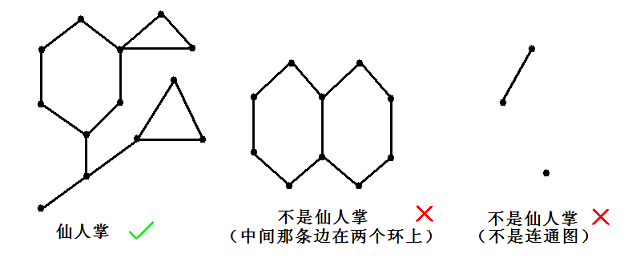# #2475. 「2018 集训队互测 Day 3」白云的旅行

#### 题目描述#### 输入格式

$1$ 行两个正整数 $n,m$ 表示城市的个数和道路的条数。保证 $n \ge 2$

#### 样例输入 1

11 11
1 2 1
2 3 1
3 4 1
4 5 1
5 6 1
6 1 1
2 7 10
3 8 20
4 9 30
5 10 40
6 11 50

#### 样例输出 1

3
2
2
2
2
2
20
40
60
80
100

#### 数据范围与提示

1 $10^5$ $1$ $m=n-1$
2 $10^5$ $998244352$ $m=n-1$
3 $8$ $998244352$
4 $10^5$ $1$ 一个点最多只在一个环中
5 $10^5$ $998244352$ 一个点最多只在一个环中
6 $500$ $998244352$
7 $3000$ $1$
8 $3000$ $998244352$
9 $10^5$ $1$
10 $10^5$ $998244352$